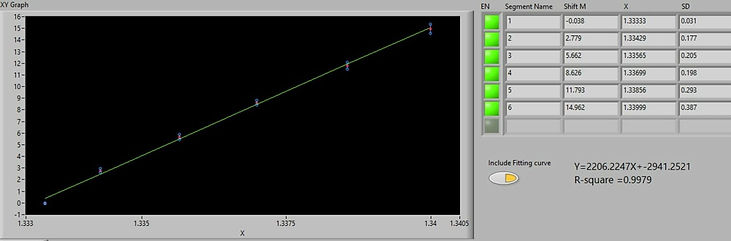## Example of Data ProcessingUnder the Data Analysis tab, data can recall during or after for rapid processing. In under to measure all the SPR shifts of interest over a long run, the sensorgram is divided in segments of interest and the SPR shift of each segment is measured against its appropriate reference.

In the case above, six saline solutions of increasing refractive index were injected successively in the sample channel inlet of the P4SPR. Each sample was measured three times in separated channels (A, B and C) as presented in different colour in the Full Sensorgram View (left panel ). The reference channel (D, not shown) had water as reference throughout the experiment. For improved data precision, the reference channel was subtracted to the sample channels and the sample channels were averaged as presented in cyan in the Segment of Interest (right panel). By moving the red and yellow cursors in the left panel, we measured the shift between the first sample solution, water, and each subsequent solution using the Segments table functions at the bottom.

The data can be uploaded and processed during the experiment thus significantly accelerating iterations.The data saved in the Segment table with the appropriate X values (e.g., refractive index, concentration) is plotted under the Graph tab. The segment data for the graph can be selected by the user. For titration curves, the software provide the slope equation and the coefficient of correlation. For an estimated KD, go in the Affinity tab to generate a value.

A titration curve and even an estimated KD can be determined within minutes cutting down data processing time significantly.